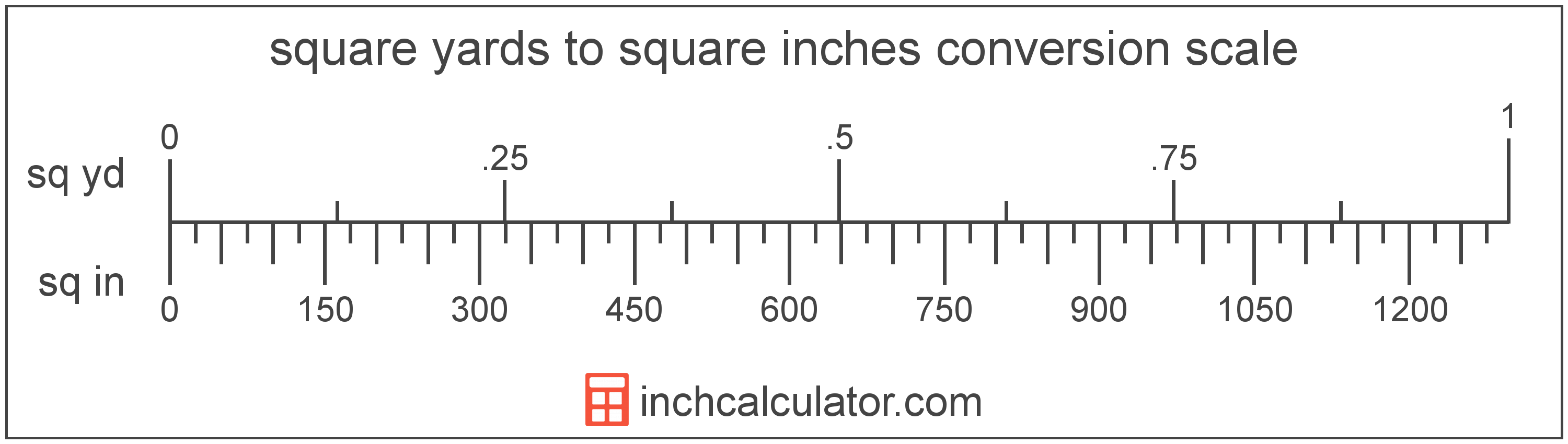# Square Inches to Square Yards Converter

Enter the area in square inches below to get the value converted to square yards.

Results in Square Yards:1,296 sq in = 1 sq yd

Do you want to convert square yards to square inches?

## How to Convert Square Inches to Square Yards

To convert a measurement in square inches to a measurement in square yards, divide the area by the following conversion ratio: 1,296 square inches/square yard.

Since one square yard is equal to 1,296 square inches, you can use this simple formula to convert:

square yards = square inches ÷ 1,296

The area in square yards is equal to the area in square inches divided by 1,296.

For example, here's how to convert 5,000 square inches to square yards using the formula above.
square yards = (5,000 sq in ÷ 1,296) = 3.858025 sq yd## What Is a Square Inch?

One square inch is equivalent to the area of a square with sides that are each 1 inch in length. One square inch is equal to 1/144 of a square foot or 1/1,296 of a square yard.

The square inch is a US customary and imperial unit of area. A square inch is sometimes also referred to as a square in. Square inches can be abbreviated as sq in, and are also sometimes abbreviated as in². For example, 1 square inch can be written as 1 sq in or 1 in².

## What Is a Square Yard?

One square yard is equivalent to the area of a square with sides that are each 1 yard in length. One square yard is roughly equal to 9 square feet or 0.836127 square meters.

The square yard is a US customary and imperial unit of area. A square yard is sometimes also referred to as a square yd. Square yards can be abbreviated as sq yd, and are also sometimes abbreviated as yd². For example, 1 square yard can be written as 1 sq yd or 1 yd².

You can use a square yards calculator to calculate the area of a space if you know its dimensions.

## Square Inch to Square Yard Conversion Table

Table showing various square inch measurements converted to square yards.
Square Inches Square Yards
1 sq in 0.000772 sq yd
2 sq in 0.001543 sq yd
3 sq in 0.002315 sq yd
4 sq in 0.003086 sq yd
5 sq in 0.003858 sq yd
6 sq in 0.00463 sq yd
7 sq in 0.005401 sq yd
8 sq in 0.006173 sq yd
9 sq in 0.006944 sq yd
10 sq in 0.007716 sq yd
20 sq in 0.015432 sq yd
30 sq in 0.023148 sq yd
40 sq in 0.030864 sq yd
50 sq in 0.03858 sq yd
60 sq in 0.046296 sq yd
70 sq in 0.054012 sq yd
80 sq in 0.061728 sq yd
90 sq in 0.069444 sq yd
100 sq in 0.07716 sq yd
200 sq in 0.154321 sq yd
300 sq in 0.231481 sq yd
400 sq in 0.308642 sq yd
500 sq in 0.385802 sq yd
600 sq in 0.462963 sq yd
700 sq in 0.540123 sq yd
800 sq in 0.617284 sq yd
900 sq in 0.694444 sq yd
1,000 sq in 0.771605 sq yd
2,000 sq in 1.5432 sq yd
3,000 sq in 2.3148 sq yd
4,000 sq in 3.0864 sq yd
5,000 sq in 3.858 sq yd
6,000 sq in 4.6296 sq yd
7,000 sq in 5.4012 sq yd
8,000 sq in 6.1728 sq yd
9,000 sq in 6.9444 sq yd
10,000 sq in 7.716 sq yd